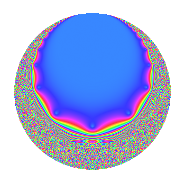Properties

 Label 20.10.a.bLevel 20 Weight 10 Character orbit 20.a Self dual Yes Analytic conductor 10.301 Analytic rank 0 Dimension 2 CM No Inner twists 1

Related objects

Newspace parameters

 Level: $$N$$ = $$20 = 2^{2} \cdot 5$$ Weight: $$k$$ = $$10$$ Character orbit: $$[\chi]$$ = 20.a (trivial)

Newform invariants

 Self dual: Yes Analytic conductor: $$10.3007167233$$ Analytic rank: $$0$$ Dimension: $$2$$ Coefficient field: $$\Q(\sqrt{79})$$ Coefficient ring: $$\Z[a_1, a_2, a_3]$$ Coefficient ring index: $$2^{4}$$ Fricke sign: $$-1$$ Sato-Tate group: $\mathrm{SU}(2)$

$q$-expansion

Coefficients of the $$q$$-expansion are expressed in terms of $$\beta = 16\sqrt{79}$$. We also show the integral $$q$$-expansion of the trace form.

 $$f(q)$$ $$=$$ $$q$$ $$+ ( -130 + \beta ) q^{3}$$ $$-625 q^{5}$$ $$+ ( -190 + 69 \beta ) q^{7}$$ $$+ ( 17441 - 260 \beta ) q^{9}$$ $$+O(q^{10})$$ $$q$$ $$+ ( -130 + \beta ) q^{3}$$ $$-625 q^{5}$$ $$+ ( -190 + 69 \beta ) q^{7}$$ $$+ ( 17441 - 260 \beta ) q^{9}$$ $$+ ( 51360 + 30 \beta ) q^{11}$$ $$+ ( 89570 + 564 \beta ) q^{13}$$ $$+ ( 81250 - 625 \beta ) q^{15}$$ $$+ ( 158010 + 348 \beta ) q^{17}$$ $$+ ( 68636 + 5160 \beta ) q^{19}$$ $$+ ( 1420156 - 9160 \beta ) q^{21}$$ $$+ ( -332730 - 6393 \beta ) q^{23}$$ $$+ 390625 q^{25}$$ $$+ ( -4966780 + 31558 \beta ) q^{27}$$ $$+ ( -3446874 - 5880 \beta ) q^{29}$$ $$+ ( 145916 - 26250 \beta ) q^{31}$$ $$+ ( -6070080 + 47460 \beta ) q^{33}$$ $$+ ( 118750 - 43125 \beta ) q^{35}$$ $$+ ( 5630690 - 59976 \beta ) q^{37}$$ $$+ ( -237764 + 16250 \beta ) q^{39}$$ $$+ ( 14886726 + 72180 \beta ) q^{41}$$ $$+ ( -5854090 - 99615 \beta ) q^{43}$$ $$+ ( -10900625 + 162500 \beta ) q^{45}$$ $$+ ( 31246650 + 42573 \beta ) q^{47}$$ $$+ ( 55968957 - 26220 \beta ) q^{49}$$ $$+ ( -13503348 + 112770 \beta ) q^{51}$$ $$+ ( 4708890 - 212532 \beta ) q^{53}$$ $$+ ( -32100000 - 18750 \beta ) q^{55}$$ $$+ ( 95433160 - 602164 \beta ) q^{57}$$ $$+ ( -46465428 - 373980 \beta ) q^{59}$$ $$+ ( 97836962 + 721440 \beta ) q^{61}$$ $$+ ( -366132350 + 1252829 \beta ) q^{63}$$ $$+ ( -55981250 - 352500 \beta ) q^{65}$$ $$+ ( -109883710 + 203139 \beta ) q^{67}$$ $$+ ( -86037132 + 498360 \beta ) q^{69}$$ $$+ ( 155603508 - 1397610 \beta ) q^{71}$$ $$+ ( -49612030 - 2047716 \beta ) q^{73}$$ $$+ ( -50781250 + 390625 \beta ) q^{75}$$ $$+ ( 32105280 + 3538140 \beta ) q^{77}$$ $$+ ( 271130888 + 70020 \beta ) q^{79}$$ $$+ ( 940619189 - 3951740 \beta ) q^{81}$$ $$+ ( -628457850 + 192957 \beta ) q^{83}$$ $$+ ( -98756250 - 217500 \beta ) q^{85}$$ $$+ ( 329176500 - 2682474 \beta ) q^{87}$$ $$+ ( -231145926 + 5421960 \beta ) q^{89}$$ $$+ ( 770018884 + 6073170 \beta ) q^{91}$$ $$+ ( -549849080 + 3558416 \beta ) q^{93}$$ $$+ ( -42897500 - 3225000 \beta ) q^{95}$$ $$+ ( 835858370 - 2902956 \beta ) q^{97}$$ $$+ ( 738022560 - 12830370 \beta ) q^{99}$$ $$+O(q^{100})$$ $$\operatorname{Tr}(f)(q)$$ $$=$$ $$2q$$ $$\mathstrut -\mathstrut 260q^{3}$$ $$\mathstrut -\mathstrut 1250q^{5}$$ $$\mathstrut -\mathstrut 380q^{7}$$ $$\mathstrut +\mathstrut 34882q^{9}$$ $$\mathstrut +\mathstrut O(q^{10})$$ $$2q$$ $$\mathstrut -\mathstrut 260q^{3}$$ $$\mathstrut -\mathstrut 1250q^{5}$$ $$\mathstrut -\mathstrut 380q^{7}$$ $$\mathstrut +\mathstrut 34882q^{9}$$ $$\mathstrut +\mathstrut 102720q^{11}$$ $$\mathstrut +\mathstrut 179140q^{13}$$ $$\mathstrut +\mathstrut 162500q^{15}$$ $$\mathstrut +\mathstrut 316020q^{17}$$ $$\mathstrut +\mathstrut 137272q^{19}$$ $$\mathstrut +\mathstrut 2840312q^{21}$$ $$\mathstrut -\mathstrut 665460q^{23}$$ $$\mathstrut +\mathstrut 781250q^{25}$$ $$\mathstrut -\mathstrut 9933560q^{27}$$ $$\mathstrut -\mathstrut 6893748q^{29}$$ $$\mathstrut +\mathstrut 291832q^{31}$$ $$\mathstrut -\mathstrut 12140160q^{33}$$ $$\mathstrut +\mathstrut 237500q^{35}$$ $$\mathstrut +\mathstrut 11261380q^{37}$$ $$\mathstrut -\mathstrut 475528q^{39}$$ $$\mathstrut +\mathstrut 29773452q^{41}$$ $$\mathstrut -\mathstrut 11708180q^{43}$$ $$\mathstrut -\mathstrut 21801250q^{45}$$ $$\mathstrut +\mathstrut 62493300q^{47}$$ $$\mathstrut +\mathstrut 111937914q^{49}$$ $$\mathstrut -\mathstrut 27006696q^{51}$$ $$\mathstrut +\mathstrut 9417780q^{53}$$ $$\mathstrut -\mathstrut 64200000q^{55}$$ $$\mathstrut +\mathstrut 190866320q^{57}$$ $$\mathstrut -\mathstrut 92930856q^{59}$$ $$\mathstrut +\mathstrut 195673924q^{61}$$ $$\mathstrut -\mathstrut 732264700q^{63}$$ $$\mathstrut -\mathstrut 111962500q^{65}$$ $$\mathstrut -\mathstrut 219767420q^{67}$$ $$\mathstrut -\mathstrut 172074264q^{69}$$ $$\mathstrut +\mathstrut 311207016q^{71}$$ $$\mathstrut -\mathstrut 99224060q^{73}$$ $$\mathstrut -\mathstrut 101562500q^{75}$$ $$\mathstrut +\mathstrut 64210560q^{77}$$ $$\mathstrut +\mathstrut 542261776q^{79}$$ $$\mathstrut +\mathstrut 1881238378q^{81}$$ $$\mathstrut -\mathstrut 1256915700q^{83}$$ $$\mathstrut -\mathstrut 197512500q^{85}$$ $$\mathstrut +\mathstrut 658353000q^{87}$$ $$\mathstrut -\mathstrut 462291852q^{89}$$ $$\mathstrut +\mathstrut 1540037768q^{91}$$ $$\mathstrut -\mathstrut 1099698160q^{93}$$ $$\mathstrut -\mathstrut 85795000q^{95}$$ $$\mathstrut +\mathstrut 1671716740q^{97}$$ $$\mathstrut +\mathstrut 1476045120q^{99}$$ $$\mathstrut +\mathstrut O(q^{100})$$

Embeddings

For each embedding $$\iota_m$$ of the coefficient field, the values $$\iota_m(a_n)$$ are shown below.

For more information on an embedded modular form you can click on its label.

Label $$\iota_m(\nu)$$ $$a_{2}$$ $$a_{3}$$ $$a_{4}$$ $$a_{5}$$ $$a_{6}$$ $$a_{7}$$ $$a_{8}$$ $$a_{9}$$ $$a_{10}$$
1.1
 −8.88819 8.88819
0 −272.211 0 −625.000 0 −10002.6 0 54415.9 0
1.2 0 12.2111 0 −625.000 0 9622.57 0 −19533.9 0
 $$n$$: e.g. 2-40 or 990-1000 Significant digits: Format: Complex embeddings Normalized embeddings Satake parameters Satake angles

Inner twists

This newform does not admit any (nontrivial) inner twists.

Atkin-Lehner signs

$$p$$ Sign
$$2$$ $$-1$$
$$5$$ $$1$$

Hecke kernels

This newform can be constructed as the kernel of the linear operator $$T_{3}^{2}$$ $$\mathstrut +\mathstrut 260 T_{3}$$ $$\mathstrut -\mathstrut 3324$$ acting on $$S_{10}^{\mathrm{new}}(\Gamma_0(20))$$.